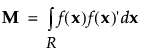Publication date: 07/30/2020

## Save X Matrix

This option saves scripts called Moments Matrix and Model Matrix that contain the moments matrix and the model matrix to the design data table. The moments matrix and the model matrix are used to calculate the Average Variance of Prediction, which appears in the Design Diagnostics section of the Design Evaluation outline. See Goos and Jones (2011). If the design is a split-plot design, a V Inverse script is also saved. The V Inverse script contains the inverse of the covariance matrix of the responses.

Caution: For a design with nominal factors, the Model Matrix saved by the Save X Matrix option is not the coding matrix used in fitting the linear model. You can obtain the coding matrix used for fitting the model by selecting the option Save Columns > Save Coding Table in the Fit Model report that you obtain when you run the Model script.

Note: You can set a preference to always save the matrix script. Select File > Preferences > Platforms > DOE. Check Save X Matrix.

#### Model Matrix

The model matrix describes the design for the experiment. The model matrix has a row for each run and a column for each term of the model specified in the Model outline. For each run, the corresponding row of the model matrix contains the coded values of the model terms:

Continuous terms are coded to span the range from -1 to 1.

Nominal terms are coded by applying the Gram-Schmidt orthogonalization procedure to the coding vectors used in fitting linear models.

Note: Coding for power analysis matches that of fitting linear models. For more information about the coding used for nominal terms in fitting linear models, see Coding for Nominal Effects in Fitting Linear Models.

#### Moments Matrix

The moments matrix is dependent upon the model effects but is independent of the design. It is defined as follows:where f(x) denotes the model effects corresponding to factor combinations of the vector of factors, x, and R denotes the design space. For more information about moments and design matrices, see Goos and Jones (2011) and Myers et al. (2009). Note that the moments matrix is called a matrix of region moments in Myers et al. (2009).

#### Scripts

From the Custom Design red triangle menu, select Save X Matrix. After the design and the table are created, in the Custom Design table, the Moments Matrix and Model Matrix scripts, and if the design is a split plot, the V Inverse script, are saved as table scripts.

Right-click and Select Edit from either the Moments Matrix, Model Matrix, or V Inverse script. The script shows the corresponding matrix. You can copy this matrix into scripts that you write.

When you run the Moments Matrix script, the log shows the number of rows in the moments matrix, called Moments.

When you run the script Model Matrix, the log displays the number of rows in the model matrix, called X.

When you run the script V Inverse, the log displays the number of rows in the inverse covariance matrix, called V Inverse.

#### Example

Follow these steps to illustrate these features:

Tip: To see the log, select View > Log (Window > Log on Mac).

1. Select DOE > Custom Design.

2. Add 3 continuous factors and click Continue.

3. Click Interactions > 2nd.

4. Click the Custom Design red triangle and select Save X Matrix.

5. Using the Default Number of Runs (12), click Make Design and then Make Table.

6. In the Table panel, right-click the Moments Matrix script and select Edit.

The script appears in a script window. The script shows the moments matrix, which is called Moments.

Figure 4.28 Moments Matrix Script7. If it is not already open, select View > Log (Window > Log on the Mac).

8. In the Table panel, click the green triangle next to the Moments Matrix script.

The number of rows appear in the log as N Row(Moments)=7.

9. In the Table panel, right-click the Model Matrix script and select Edit.

The script appears in a script window. The script shows the model matrix, which is called X.

10. Click Run.

The number of rows appears in the log as N Row(X)=12.

11. To view the Model Matrix as a data table, add these lines to the script:

`dt = New Table( "Model Matrix" );`
`dt << Set Matrix( X );`

12. Click Run.